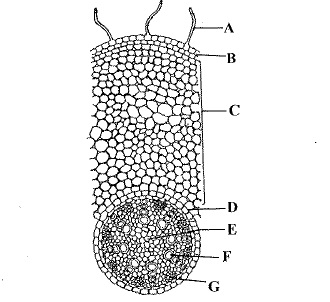• Subject:

...

• Topic:

...

In the given diagram of section of root of maize, different parts have been indicated by alphabets. Choose the answer in which these alphabets have been correctly matched with the parts which they indicate.(a) A = Phloem, B = Xylem, C = Cortex, D = Endodermis, E = Pith, F = Root hair, G = Epidermis

(b) A = Pith, B = Root hair, C = Epiblema, D = Cortex, E = Endodermis, F = Phloem, G = Xylem

(c) A = Root hair, B = Epiblema, C = Cortex, D = Endodermis, E = Pith, F = Xylem, G = Phloem

(d) A = Epiblema, B = Root hair, C = Vascular bundle, D = Cortex, E = Phloem, F = Xylem, G = Pith